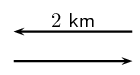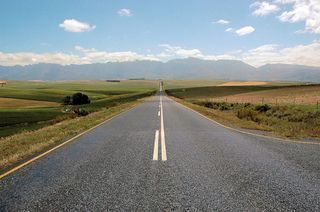We think you are located in United States. Is this correct?

# 21.3 Speed and velocity

## 21.3 Speed and velocity (ESAGW)

Average speed

Average speed is the distance ($$D$$) travelled divided by the time $$\Delta t$$) taken for the journey.

Quantity: average speed ($${v}_{av}$$) Unit name: metre per second Unit symbol: $$\text{m·s^{-1}}$$

Average velocity

Average velocity is the change in position of a body divided by the time it took for the displacement to occur.

Quantity: average velocity ($$\vec{v}_{av}$$) Unit name: metre per second Unit symbol: $$\text{m·s^{-1}}$$

Before moving on review the difference between distance and displacement. Sometimes the average speed can be a very big number while the average velocity is zero.

Average velocity is the rate of change of position. It tells us how much an object's position changes per unit of time. Velocity is a vector. We use the symbol $$\vec{v}_{av}$$ for average velocity. If we have a displacement of $$\Delta \vec{x}$$ and a time taken of $$\Delta t$$, $$\vec{v}_{av}$$ is then defined as:

\begin{align*} \text{average velocity (in } \text{m·s$^{-1}$} \text{) }& = \frac{\text{change in position (in } \text{m} \text{)}}{\text{change in time (in } \text{s} \text{)}} \\ \vec{v}_{av} & = \frac{\Delta \vec{x}}{\Delta t} \end{align*}

Velocity can be positive or negative. A positive velocity points in the direction you chose as positive in your coordinate system. A negative velocity points in the direction opposite to the positive direction.

Average speed (symbol $${v}_{av}$$) is the distance travelled ($$D$$) divided by the time taken ($$\Delta t$$) for the journey. Distance and time are scalars and therefore speed will also be a scalar. Speed is calculated as follows:

\begin{align*} \text{average speed (in } \text{m·s$^{-1}$} \text{) }& = \frac{\text{change in position (in } \text{m} \text{)}}{\text{change in time (in } \text{s} \text{)}} \\ v_{av} & = \frac{D}{\Delta t} \end{align*}

## Worked example 1: Average speed and average velocity

James walks $$\text{2}$$ $$\text{km}$$ away from home in $$\text{30}$$ minutes. He then turns around and walks back home along the same path, also in $$\text{30}$$ minutes. Calculate James' average speed and average velocity.### Identify what information is given and what is asked for

The question explicitly gives

• the distance and time out ($$\text{2}$$ $$\text{km}$$ in $$\text{30}$$ minutes)

• the distance and time back ($$\text{2}$$ $$\text{km}$$ in $$\text{30}$$ minutes)

### Check that all units are SI units.

The information is not in SI units and must therefore be converted.

To convert $$\text{km}$$ to $$\text{m}$$, we know that:

\begin{align*} \text{1}\text{ km} & = \text{1 000}\text{ m} \\ \therefore \text{2}\text{ km} & = \text{2 000}\text{ m} \text{ (multiply both sides by } 2 \end{align*}

Similarly, to convert $$\text{30}$$ minutes to seconds,

\begin{align*} \text{1}\text{ min} & = \text{60}\text{ s} \\ \therefore \text{30}\text{ min} & = \text{1 800}\text{ s} \text{ (multiply both sides by } 20 \end{align*}

### Determine James' displacement and distance.

James started at home and returned home, so his displacement is $$\text{0}$$ $$\text{m}$$.

$$\Delta \vec{x} = \text{0}\text{ m}$$

James walked a total distance of $$\text{4 000}$$ $$\text{m}$$ ($$\text{2 000}$$ $$\text{m}$$ out and $$\text{2 000}$$ $$\text{m}$$ back).

$$D = \text{4 000}\text{ km}$$

### Determine his total time.

James took $$\text{1 800}$$ $$\text{s}$$ to walk out and $$\text{1 800}$$ $$\text{s}$$ to walk back.

$$\Delta t = \text{3 600}\text{ s}$$

### Determine his average speed

\begin{align*} v_{av} & = \frac{D}{\Delta t} \\ & = \frac{\text{4 000}\text{ m}}{\text{3 600}\text{ km}} \\ & = \text{1,11}\text{ m·s$^{-1}$} \end{align*}

### Determine his average velocity

\begin{align*} \vec{v}_{av} & = \frac{\Delta \vec{x}}{\Delta t} \\ & = \frac{\text{0}\text{ m}}{\text{3 600}\text{ km}} \\ & = \text{0}\text{ m·s$^{-1}$} \end{align*}

### Differences between speed and velocity (ESAGX)

The differences between speed and velocity can be summarised as:

 Speed Velocity 1. depends on the path taken 1. independent of path taken 2. always positive 2. can be positive or negative 3. is a scalar 3. is a vector 4. no dependence on direction and so is only positive 4. direction can be determined from the sign convention used (i.e. positive or negative)

Additionally, an object that makes a round trip, i.e. travels away from its starting point and then returns to the same point has zero velocity but travels at a non-zero speed.

## Displacement and related quantities

Textbook Exercise 21.3

Bongani has to walk to the shop to buy some milk. After walking $$\text{100}$$ $$\text{m}$$, he realises that he does not have enough money, and goes back home. If it took him two minutes to leave and come back, calculate the following:

1. How long was he out of the house (the time interval $$\Delta t$$ in seconds)?

2. How far did he walk (distance ($$D$$)?

3. What was his displacement ($$\Delta \vec{x}$$)?

4. What was his average velocity (in $$\text{m·s^{-1}}$$)?

5. What was his average speed (in $$\text{m·s^{-1}}$$)?

Solution not yet available

Bridget is watching a straight stretch of road from her classroom window. She can see two poles which she earlier measured to be $$\text{50}$$ $$\text{m}$$ apart. Using her stopwatch, Bridget notices that it takes $$\text{3}$$ $$\text{s}$$ for most cars to travel from the one pole to the other.

1. Using the equation for velocity $$\left({\vec{v}}_{av} = \frac{\Delta \vec{x}}{\Delta t}\right)$$, show all the working needed to calculate the velocity of a car travelling from the left to the right.

2. If Bridget measures the velocity of a red Golf to be $$-\text{16,67}$$ $$\text{m·s^{-1}}$$, in which direction was the Golf travelling?

3. Bridget leaves her stopwatch running, and notices that at $$t = \text{5,0}\text{ s}$$, a taxi passes the left pole at the same time as a bus passes the right pole. At time $$t = \text{7,5}\text{ s}$$ the taxi passes the right pole. At time $$t = \text{9,0}\text{ s}$$, the bus passes the left pole.

How long did it take the taxi and the bus to travel the distance between the poles? (Calculate the time interval ($$\Delta t$$

4. What was the average velocity of the taxi and the bus?

5. What was the average speed of the taxi and the bus?

6. What was the average speed of taxi and the bus in $$\text{km·h^{-1}}$$?

Solution not yet available

A rabbit runs across a freeway. There is a car, $$\text{100}$$ $$\text{m}$$ away travelling towards the rabbit.

1. If the car is travelling at $$\text{120}$$ $$\text{km·h^{-1}}$$, what is the car's speed in $$\text{m·s^{-1}}$$.

2. How long will it take the a car to travel $$\text{100}$$ $$\text{m}$$?

3. If the rabbit is running at $$\text{10}$$ $$\text{km·h^{-1}}$$, what is its speed in $$\text{m·s^{-1}}$$?

4. If the freeway has $$\text{3}$$ lanes, and each lane is $$\text{3}$$ $$\text{m}$$ wide, how long will it take for the rabbit to cross all three lanes?

5. If the car is travelling in the furthermost lane from the rabbit, will the rabbit be able to cross all $$\text{3}$$ lanes of the freeway safely?

Solution not yet available

## An exercise in safety

Divide into groups of $$\text{4}$$ and perform the following investigation. Each group will be performing the same investigation, but the aim for each group will be different.

1. Choose an aim for your investigation from the following list and formulate a hypothesis:

• Do cars travel at the correct speed limit?

• Is is safe to cross the road outside of a pedestrian crossing?

• Does the colour of your car determine the speed you are travelling at?

• Any other relevant question that you would like to investigate.

2. On a road that you often cross, measure out $$\text{50}$$ $$\text{m}$$ along a straight section, far away from traffic lights or intersections.

3. Use a stopwatch to record the time each of $$\text{20}$$ cars take to travel the $$\text{50}$$ $$\text{m}$$ section you measured.

4. Design a table to represent your results. Use the results to answer the question posed in the aim of the investigation. You might need to do some more measurements for your investigation. Plan in your group what else needs to be done.

• Aim and Hypothesis

• Apparatus

• Method

• Results

• Discussion

• Conclusion

1. How many cars took less than $$\text{3}$$ $$\text{s}$$ to travel $$\text{50}$$ $$\text{m}$$?
2. What was the shortest time a car took to travel $$\text{50}$$ $$\text{m}$$?
3. What was the average time taken by the $$\text{20}$$ cars?
4. What was the average speed of the $$\text{20}$$ cars?
5. Convert the average speed to $$\text{km·h^{-1}}$$.